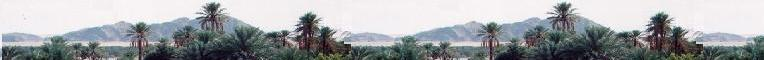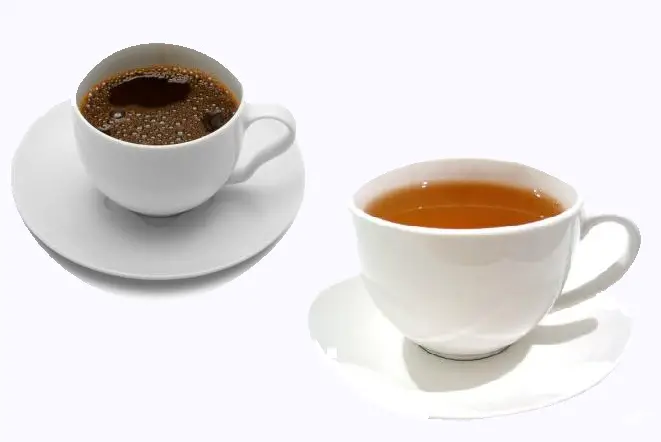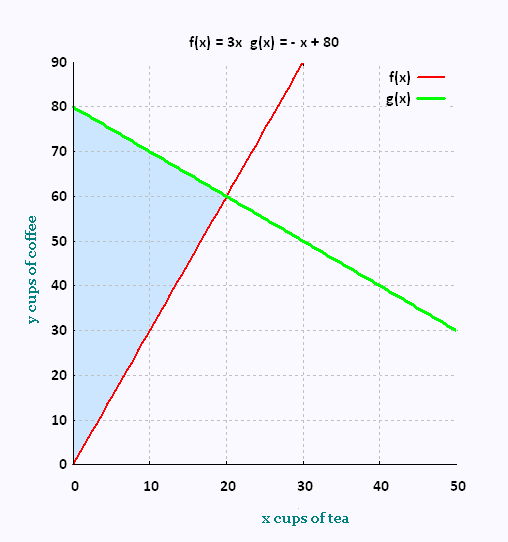# Mathematics 2: Linear optimization Examples

### Coffee shopIn a coffee shop, we serve tea and coffee. A cup of tea costs 3$and a cup of coffee costs 2$. There are no more than 80 customers that take the same beverage. There are at least three times more customers choosing coffee. The number of cups is limited to 90 for coffee and 5o for tea.

How many cups it is possible to serve to maximise the profit?

x number of cups of tea
y number of cups of coffee

• There are no more than 80 customers that take the same beverage: That is:

No customer for tea : x = 0 → y ≤ 80
No customer for coffee : y = 0 → x ≤ 80
Therefore x + y ≤ 80

• There are at least (≥) three times more customers choosing coffee. Therefore y ≥ 3 x

• x < 50
• y < 90• Function objective: Z (\$) = 3 x + 2 y (profit to maximize)

According to the polygon of constraints, we have:

x = 0, y = 0 → Z = 0 + 0 = 0

x = 0, y = 80 → Z = 0 + 160 = 160

x = 20 , y = 60 → Z = 60 + 120 = 180

Z max = 180, therefore the unique solution is x = 20 (cups of tea), and y = 60 (cups of coffee).Web ScientificSentence

 chimie labs | scientific sentence | java | php | green cat | contact |